## FLAC3D New 3D Liquefaction Constitutive Model

### Background

Fully nonlinear methods can be formulated in terms of effective stresses and the fluid-mechanical interaction behaviors can be simulated before, during, and following dynamic shaking. Multi-dimensional nonlinear constitutive models are being used increasingly to simulate the phenomenon of liquefaction for sand-like geotechnical materials in earthquake engineering practice, e.g., from the relatively simple uncoupled models like the cyclic-counting Dames & Moore model (Dawson et al. 2001) to the relatively complex effective stress-based models like the PDMY02 model (Elgamal et al. 2003), DM04 (Sanisand) model (Dafalias & Manzari 2004) model, UBCSand model (Beaty & Byrne 2011), and PM4Sand model (Boulanger & Ziotopoulou 2015), to name some. While all of the aforementioned models have merit they also have some notable limitations and many of them are not formulated for 3D modeling.

### P2P-Sand: An Introduction

Itasca is pleased to announce P2P-Sand (Practical Two-Surface Plasticity model for Sands), our new practice-friendly 3D liquefaction constitutive model for FLAC3D based on the DM04 (Sanisand) model (Dafalias & Manzari, 2004). By revising some formula without destroying the conciseness of the original DM04 (Sanisand) model, the modifications improve the comparative results between model simulations and various laboratory and field observations with various initial and loading conditions. In addition, Itasca's new liquefaction model embraces an easier and more practical calibration procedure in terms of in-situ data, instead of experimental data, greatly reducing the laboriousness of calibration.

Itasca's enhanced model:

• retains the general 3D formulation, considering Lode angle effect, and thus can be used for general 3D boundary value problems
• switches all void-ratio related formula, including the elastic modulus, plastic hardening modulus, and critical state, to be relative density related
• requires only the initial soil relative density (Dr) and initial stresses to match the semi-empirical relation of CRR versus some in-situ parameter such as (N1)60 or qc1N when using the default parameters*
• preserves the feature that one set of model constants is used to simulate different responses with different initial relative densities and initial stress states

The following table compares a number of factors for selected liquefaction constitutive models.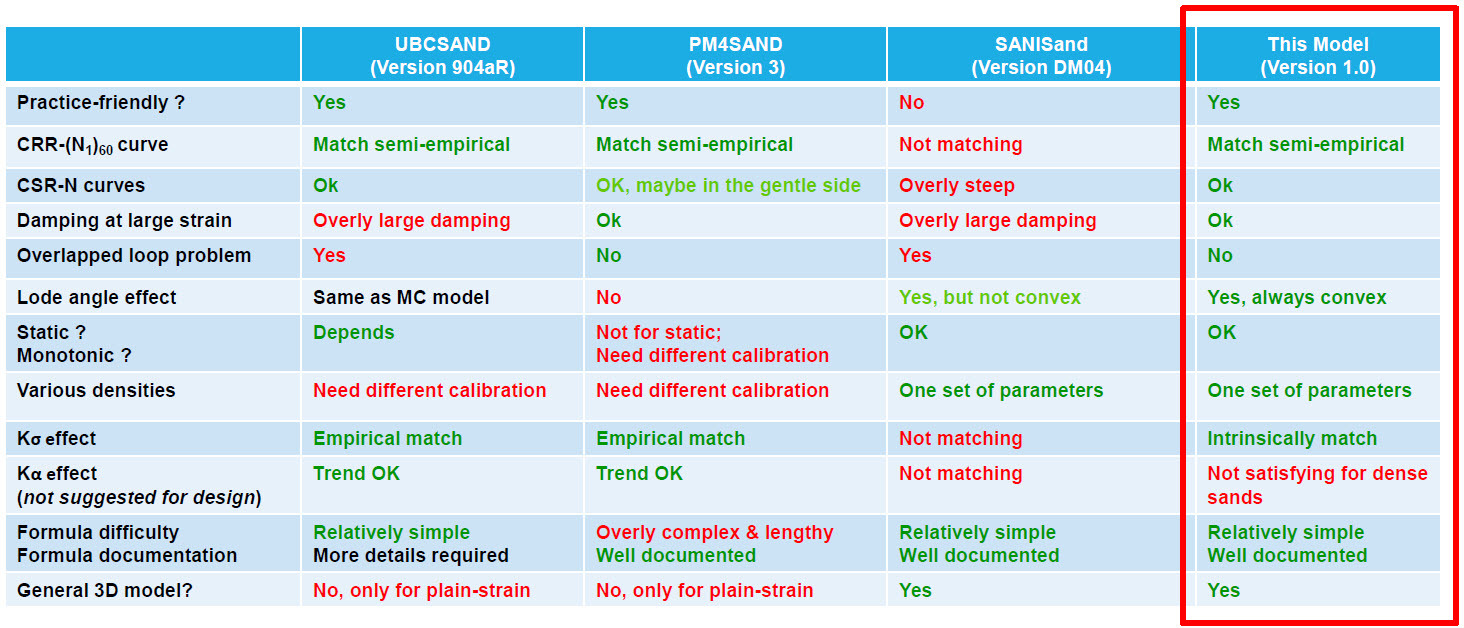### Validation

Single-zone FLAC3D models were used to test and evaluate this new liquefaction constitutive model considering the following:

• CRR versus (N1)60 and number of cycles
• Modulus reduction and damping
• Empirical Kσ curves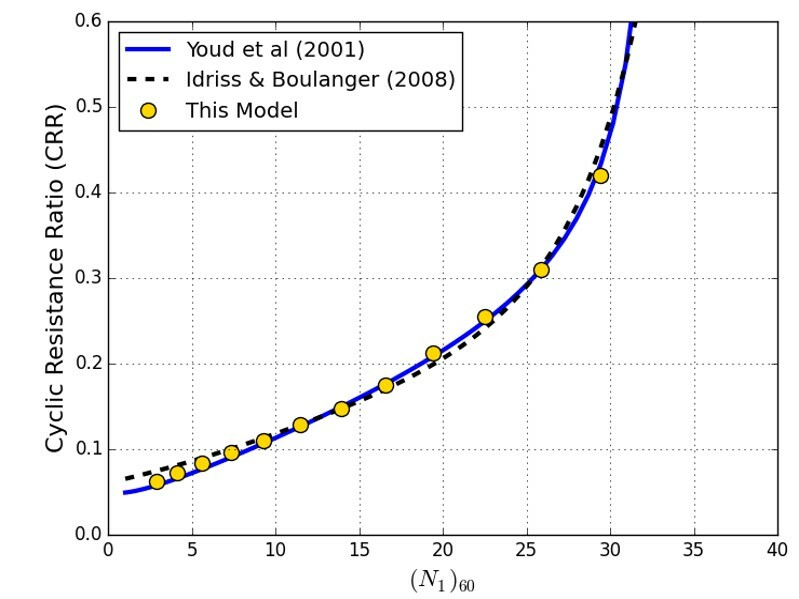Values of CRR predicted by the model and compare very well to semi-empirical curve (left); as do curves of CSR versus number of cycles in undrained DSS cyclic simulations (right).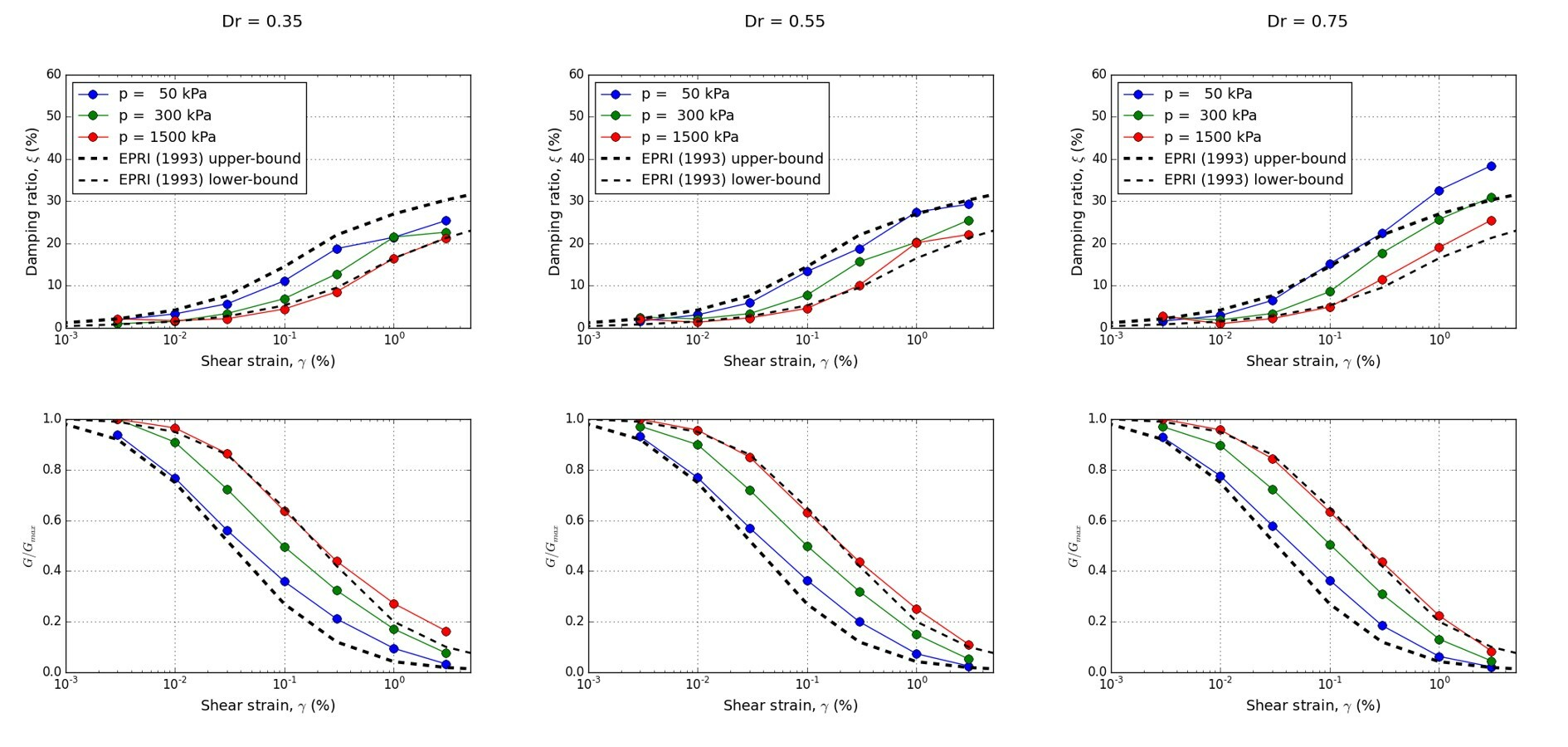Model results fall within a reasonable range of G/Gmax ratios from constant mean pressure drained triaxial simulations for sands with and Dr = 35%, 55%, and 75%.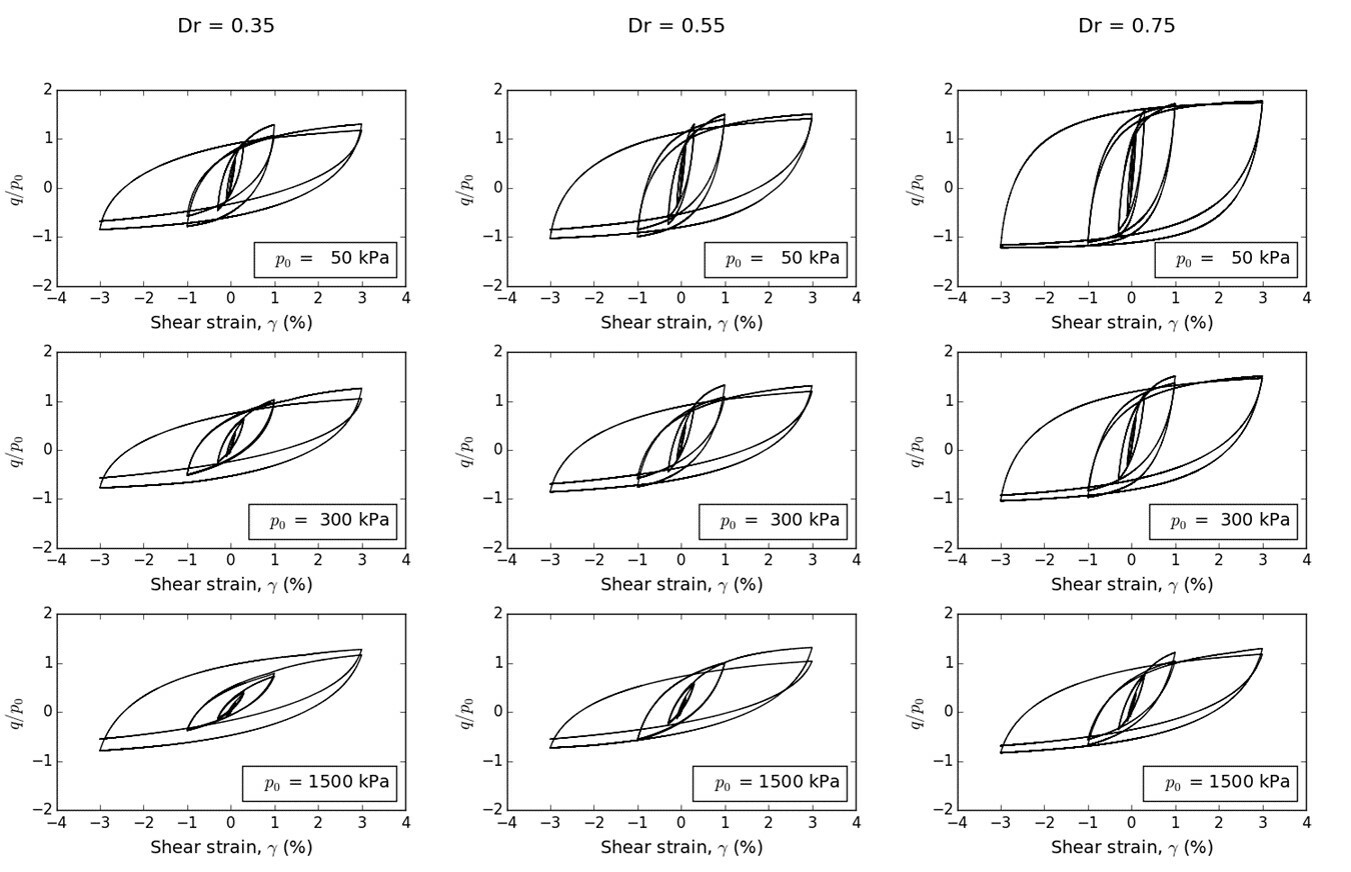Models are well behaved given damping ratios from constant mean pressure drained triaxial simulations for sands with and Dr = 35%, 55%, and 75% and Po = 50 kPa, 300 kPa, and 1500 kPa.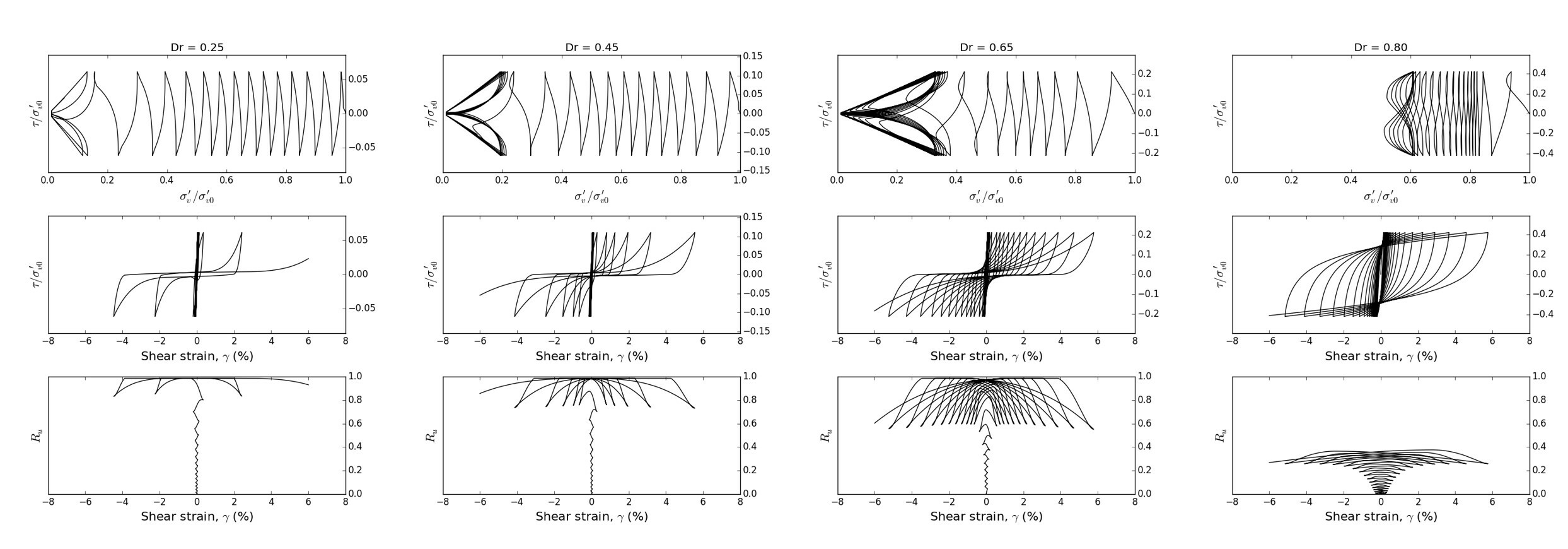Undrained cyclic DSS simulations for initial relative densities of 25%, 45%, 65%, and 80%.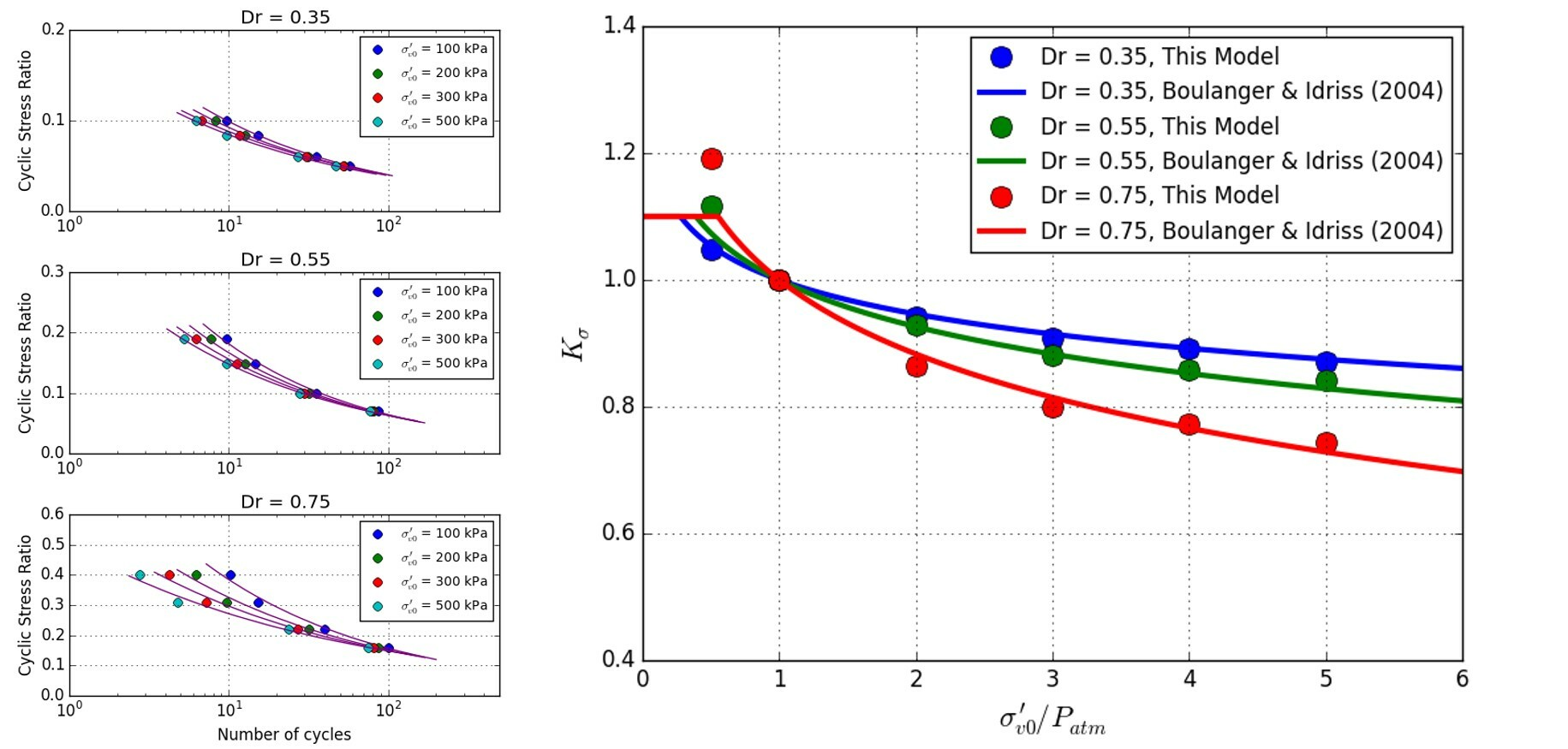Model results compare very well with Empirical Kσ curves (Boulanger and Idriss, 2004) for a range of overburden vertical effective stresses.

### Some Examples

The following engineering and laboratory scale simulations using the new liquefaction model are performing very well and behaving as expected.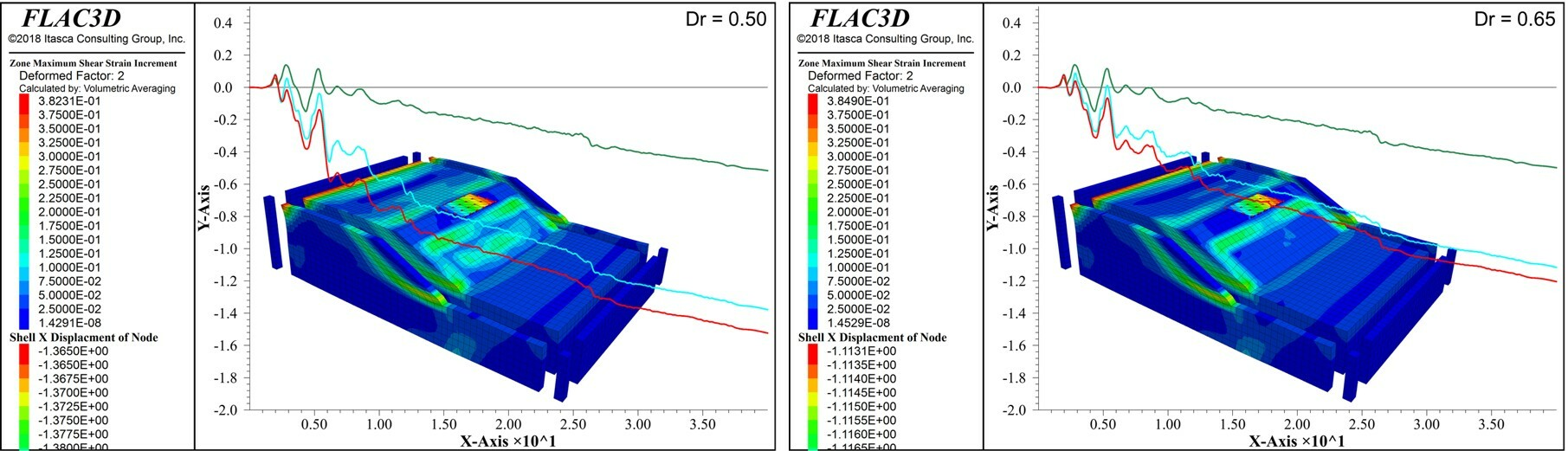FLAC3D dynamic simulation of a wharf composed of structural elements resting on a slope subjected to shaking. Itasca's new liquefaction constitutive model was used for Dr = 50% and 65%.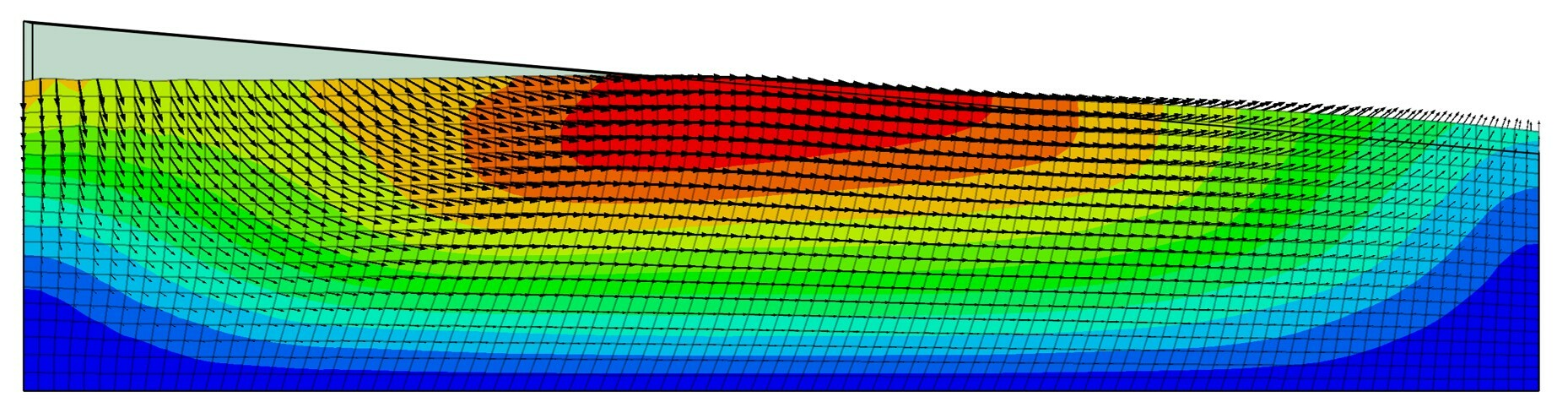Dynamic simulation of a centrifuge model showing total displacement vectors and contours of vertical displacement. The model has deformed replicating what was observed with the physical centrifuge models.

For more details about Itasca's new liquefaction model, P2P-Sand, please see the following paper:

Cheng, Zhao (2018). "A practical 3D bounding surface plastic sand model for geotechnical earthquake engineering application," Geotechnical Earthquake Engineering and Soil Dynamics (GEESD) Congress V, June 10-13, 2018, Austin, Texas, United States.